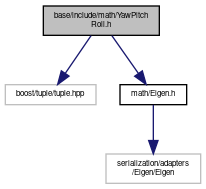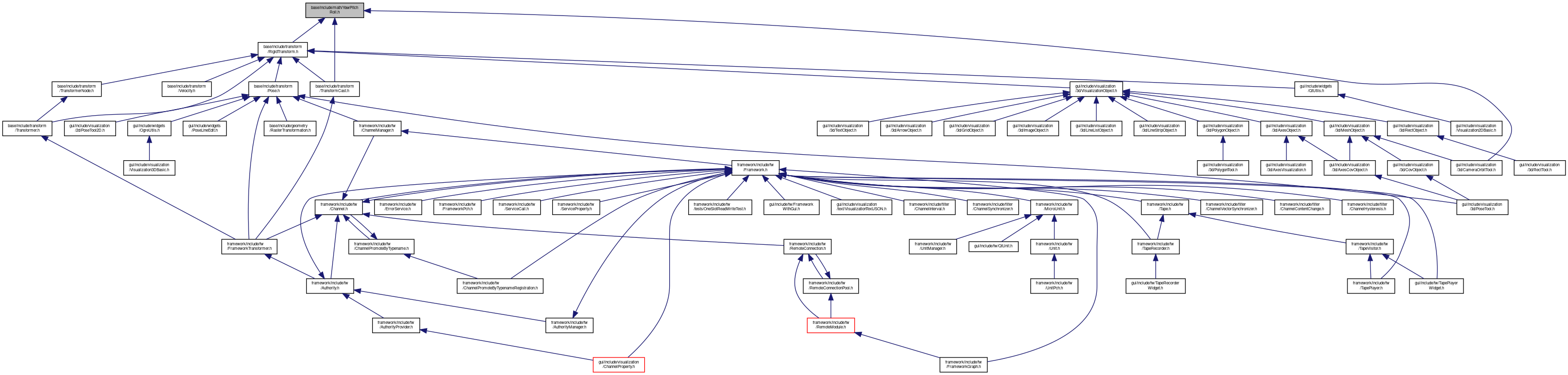MIRA
YawPitchRoll.h File Reference

Conversion from yaw, pitch and roll to Quaternion and vice versa. More...

`#include <boost/tuple/tuple.hpp>`
`#include <math/Eigen.h>`
Include dependency graph for YawPitchRoll.h:This graph shows which files directly or indirectly include this file:Go to the source code of this file.

## Namespaces

mira
specialize cv::DataType for our ImgPixel and inherit from cv::DataType<Vec>

Eigen

## Functions

template<typename Derived >
Eigen::Matrix< typename
Eigen::MatrixBase< Derived >
::Scalar, 3, 1 >
eulerAngles (const Eigen::MatrixBase< Derived > &mat, typename Eigen::MatrixBase< Derived >::Index a0, typename Eigen::MatrixBase< Derived >::Index a1, typename Eigen::MatrixBase< Derived >::Index a2)
Returns the Euler-angles of the rotation matrix mat using the convention defined by the triplet (a0,a1,a2) More...

template<typename T >
Eigen::Quaternion< T > quaternionFromYawPitchRoll (T yaw, T pitch, T roll)
Converts yaw, pitch and roll angles to a quaternion. More...

template<typename T >
Eigen::Quaternion< T > quaternionFromYawPitchRoll (const boost::tuples::tuple< T, T, T > &ypr)
Same as the above method, that takes the yaw, pitch, roll angles as 3-tuple. More...

template<typename T >
void quaternionCovFromYawPitchRollCov (const Eigen::Matrix< T, 6, 6 > &eulerCovariance, float yaw, float pitch, float roll, Eigen::Quaternion< T > &oOrientation, Eigen::Matrix< T, 7, 7 > &oCovariance)
Converts 6x6 dimensional covariance matrix (3D + yaw, pitch and roll) angles to a 7x7 dimensional quaternion covariance matrix (3D + Quaternion) Our convention for the order of rotations is as follows:

1. yaw around the Z-Axis (pointing upwards)
2. pitch around the Y-Axis (pointing left)
3. roll around the X-Axis (pointing forward)
More...

template<typename T >
Eigen::Matrix< T, 7, 7 > quaternionCovFromYawPitchRollCov (const Eigen::Matrix< T, 6, 6 > &eulerCovariance, float yaw, float pitch, float roll)
Converts 6x6 dimensional covariance matrix (3D + yaw, pitch and roll) angles to a 7x7 dimensional quaternion covariance matrix (3D + Quaternion) Our convention for the order of rotations is as follows:

1. yaw around the Z-Axis (pointing upwards)
2. pitch around the Y-Axis (pointing left)
3. roll around the X-Axis (pointing forward)
More...

template<typename T >
Eigen::Matrix< T, 7, 7 > quaternionCovFromYawPitchRollCov (const Eigen::Matrix< T, 6, 6 > &eulerCovariance, const Eigen::Quaternion< T > &rotation)
Same as the above method, that takes the orientation as yaw,pitch,roll angles. More...

template<typename T >
boost::tuples::tuple< T, T, T > quaternionToYawPitchRoll (const Eigen::Quaternion< T > &q)
Converts a quaternion back to yaw, pitch, roll angles. More...

template<typename T >
Eigen::Matrix< T, 6, 6 > quaternionCovToYawPitchRollCov (const Eigen::Matrix< T, 7, 7 > &covariance, const Eigen::Quaternion< T > &q)
Converts a 7x7 dimensional quaternion covariance (3D + Quaternion) back to a 6x6 dimensional euler covariance (3D + Yaw, Pitch, Roll). More...

template<typename T >
Eigen::Matrix< T, 3, 3 > matrixFromYawPitchRoll (T yaw, T pitch, T roll)
Converts yaw, pitch and roll angles to a rotation matrix. More...

template<typename T >
Eigen::Matrix< T, 3, 3 > matrixFromYawPitchRoll (const boost::tuples::tuple< T, T, T > &ypr)
Same as the above method, that takes the yaw,pitch,roll angles as 3-tuple. More...

template<typename T >
boost::tuples::tuple< T, T, T > matrixToYawPitchRoll (const Eigen::Matrix< T, 3, 3 > &r)
Converts a rotation matrix back to yaw, pitch, roll angles. More...

template<typename T >
void derivMatricesFromYawPitchRoll (T yaw, T pitch, T roll, Eigen::Matrix< T, 3, 3 > &oR_dyaw, Eigen::Matrix< T, 3, 3 > &oR_dpitch, Eigen::Matrix< T, 3, 3 > &oR_droll)
Returns the 3 derivates of the above rotation matrix, i.e. More...

## Detailed Description

Conversion from yaw, pitch and roll to Quaternion and vice versa.

Date
2011/05/06